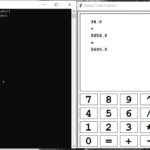## Calculator With GUI In Python With Source Code

The Calculator With GUI In Python is a simple project developed using Python. The project contains the numbers, operators, and signs like in a normal calculator. So, the user cannot enter the number themselves, they can just click on the numbers they want...

Type : Project

File Size : 2.2 KB

Please click the button below to download the ZIP File.# Similar Projects and Reports

#### GUI CALCULATOR IN PYTHON WITH SOURCE CODE

The GUI Calculator In Python is a simple project developed using Python. The project contains the numbers, operators, and sign like in normal calculator. So, the user cannot enter the number themselves, they can just click on the numbers they want in the ...

#### Calculator With GUI In Python With Source Code

The Calculator With GUI In Python is a simple project developed using Python. The project contains the numbers, operators, and signs like in a normal calculator. So, the user cannot enter the number themselves, they can just click on the numbers they want...

#### Python Calculator In GUI With Source Code

The Python Calculator In GUI is a simple project developed using Python. The project contains the numbers, operators, and signs like on a normal calculator. Here, the user can just click on the numbers they want in the calculations. Hence, the user can us...

#### CALCULATOR IN PYTHON WITH SOURCE CODE

This Calculator project In Python is a simple project developed using Python. The project contains the numbers, operators, and sign like in normal calculator. So, the user can enter any number they want in the calculations. Hence, the user can use a simpl...

#### Simple Calculator In GUI Python With Source Code

This Simple Calculator In GUI is a simple project developed using Python. The project contains the numbers, operators, and signs like on a normal calculator. Here, the user can just click on the numbers they want in the calculations. Hence, the user can u...

#### Simple GUI Calculator In Python With Source Code

The Simple GUI Calculator In Python is a simple project developed using Python. The project contains the numbers, operators, and signs like in a normal calculator. So, the user cannot enter the number themselves, they can just click on the numbers they wa...

Visit code-projects.org to download thousands of other free projects